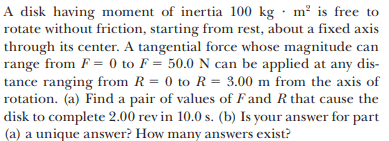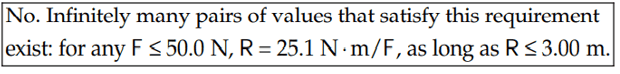# Infinite number of pairs of Force and distance R from AoR

• ChiralSuperfields
In summary, the conversation discusses the concept of infinity in relation to a mathematical model of Newtonian mechanics. There is a debate about whether or not there is an infinite number of solutions in physical reality, and it is noted that infinity is a mathematical concept and not a physical one. It is also mentioned that there may be an infinite number of points on a line, and the idea of dividing the line and assigning finite values to each point is questioned. It is suggested that the seeming contradiction could be resolved by considering the seamless and continuous nature of combining tangential force and radius. The idea of an infinite number of positions in space is also discussed, with the conclusion that there is no known perfect mathematical model of physical reality.

#### ChiralSuperfields

Homework Statement
Pls see below
Relevant Equations
Pls see below
For part (b),The solution isHowever, is there really an infinite number of pairs physically speaking? It would be very hard, say, vary the force applied by ##0.0000001N## for example.

Many thanks!

Callumnc1 said:
Homework Statement:: Pls see below
Relevant Equations:: Pls see below

For part (b),
View attachment 322694

The solution is
View attachment 322695

However, is there really an infinite number of pairs physically speaking? It would be very hard, say, vary the force applied by ##0.0000001N## for example.

Many thanks!
Infinity is a mathematical concept. It is possible that there is no such thing as infinity in the physical world. Infinitely many pairs of numbers satisfy the equation.

•ChiralSuperfields
haruspex said:
Infinity is a mathematical concept. It is possible that there is no such thing as infinity in the physical world. Infinitely many pairs of numbers satisfy the equation.
Ahh ok thank you @haruspex ! I guess this is matter of philosophy

Callumnc1 said:
However, is there really an infinite number of pairs physically speaking? It would be very hard, say, vary the force applied by ##0.0000001N## for example.
If you draw a F versus R graph, a straight sloped line will form.
That line contains all the possible combinations of F and R that induce the same moment of 25.1 N-m shown in the response.
How many points ((F,R)pairs) can be located alone that line?

https://en.m.wikipedia.org/wiki/Point_(geometry)

https://en.m.wikipedia.org/wiki/Line_(geometry)

I believe that we create that apparent contradiction by dividing our line and assigning a finite value to each point.
In reality, the way in which the tangential force and the radius can be combined to result in a unique value of moment or torque is seamless or continuos.

Following the same reasoning, for a fixed value of radius, the magnitude of tangential force that can be applied (for example, when trying to loosen a rebelious nut with a wrench) can continuosly change from a minimum to a maximum value.

•ChiralSuperfields
Callumnc1 said:
However, is there really an infinite number of pairs physically speaking? It would be very hard, say, vary the force applied by ##0.0000001N## for example.
If you think that's very hard (physically speaking), just imagine how hard it would be to mount a 100-kg disk on a fixed axle of zero diameter as implied by the stated ability to apply a force "at any distance ranging from R = 0 to R = 3.00 m from the axis". It boggles the mind.

••Tom.G, ChiralSuperfields, erobz and 2 others
Callumnc1 said:
Ahh ok thank you @haruspex ! I guess this is matter of philosophy
If you want to propose that there are only a finite number of solutions, then please list them or describe them.

••ChiralSuperfields and kuruman
PeroK said:
If you want to propose that there are only a finite number of solutions, then please list them or describe them.
Conversely, if you insist on there being an infinite number of positions in space, prove that.

•ChiralSuperfields
haruspex said:
Conversely, if you insist on there being an infinite number of positions in space, prove that.
That's inherent in the mathematical model of Newtonian mechanics.

That said, a case could be made for the finiteness of solutions experimentally, if not theoretically.

•ChiralSuperfields
PeroK said:
That's inherent in the mathematical model of Newtonian mechanics.
Right, as I wrote in post #2, infinity is a mathematical concept, not a physical one.

•ChiralSuperfields
haruspex said:
Right, as I wrote in post #2, infinity is a mathematical concept, not a physical one.
It must be both.

•ChiralSuperfields
PeroK said:
It must be both.
There is no known mathematical model of physical reality which is believed to be perfect.

•ChiralSuperfields
Lnewqban said:
If you draw a F versus R graph, a straight sloped line will form.
That line contains all the possible combinations of F and R that induce the same moment of 25.1 N-m shown in the response.
How many points ((F,R)pairs) can be located alone that line?

https://en.m.wikipedia.org/wiki/Point_(geometry)

https://en.m.wikipedia.org/wiki/Line_(geometry)

I believe that we create that apparent contradiction by dividing our line and assigning a finite value to each point.
In reality, the way in which the tangential force and the radius can be combined to result in a unique value of moment or torque is seamless or continuos.

Following the same reasoning, for a fixed value of radius, the magnitude of tangential force that can be applied (for example, when trying to loosen a rebelious nut with a wrench) can continuosly change from a minimum to a maximum value.

There will be an infinite number points ((F,R)pairs) located alone that line.

Many thanks!

kuruman said:
If you think that's very hard (physically speaking), just imagine how hard it would be to mount a 100-kg disk on a fixed axle of zero diameter as implied by the stated ability to apply a force "at any distance ranging from R = 0 to R = 3.00 m from the axis". It boggles the mind.

PeroK said:
If you want to propose that there are only a finite number of solutions, then please list them or describe them.
haruspex said:
Conversely, if you insist on there being an infinite number of positions in space, prove that.
PeroK said:
That's inherent in the mathematical model of Newtonian mechanics.

That said, a case could be made for the finiteness of solutions experimentally, if not theoretically.
haruspex said:
Right, as I wrote in post #2, infinity is a mathematical concept, not a physical one.
PeroK said:
It must be both.
haruspex said:
There is no known mathematical model of physical reality which is believed to be perfect.
Thank you for your replies @PeroK and @haruspex !